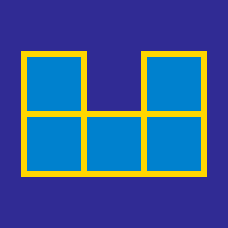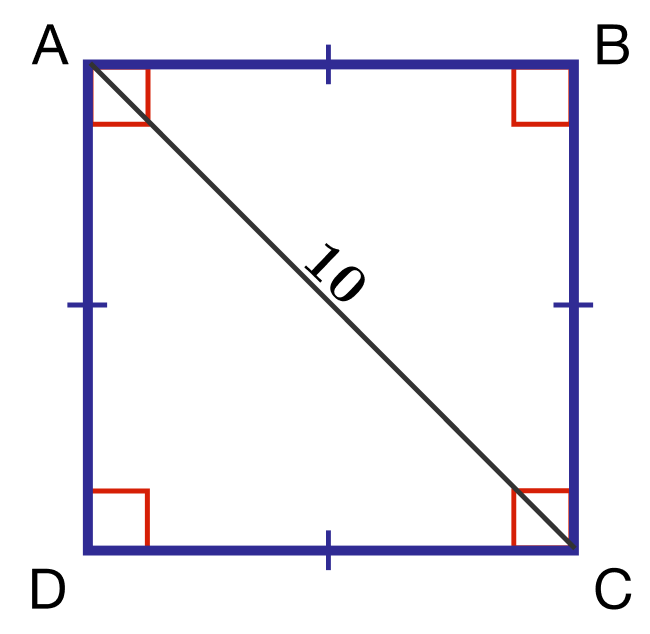Basic Mathematics

# Length and Area - Problem Solving

The width of a rectangular conference room table is $3 \mbox{ ft}$ and the area of the conference table's surface is $45 \mbox{ ft}^2$. What is the length of the conference table (in $\mbox{ft}$)?

A square has a side length of 8 inches. What is the area (in inches$^2$) of the square?

$ABCD$ is a rectangle with $AB =200$ and $BC = 480$. $E$ is a point on $CD$ (possibly extended) such that $AE=EC$. What is the length of $DE$?

$ABCD$ is a quadrilateral with $\angle ADC = \angle ACD$, $\angle ACB = \angle ABC$ and $CD = 8$. If triangles $ADC$ and $ABC$ have perimeters $58$ and $69$, respectively, what is the value of $BC$?If $\overline{AC}=10$, what is the area of square $ABCD$?

×